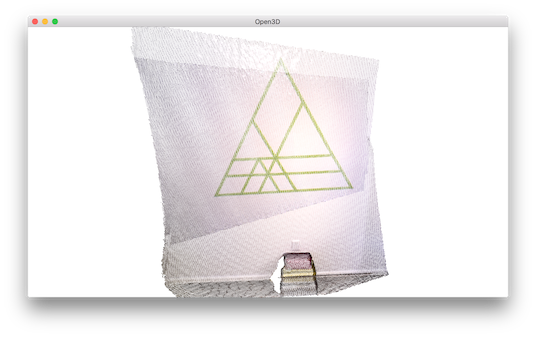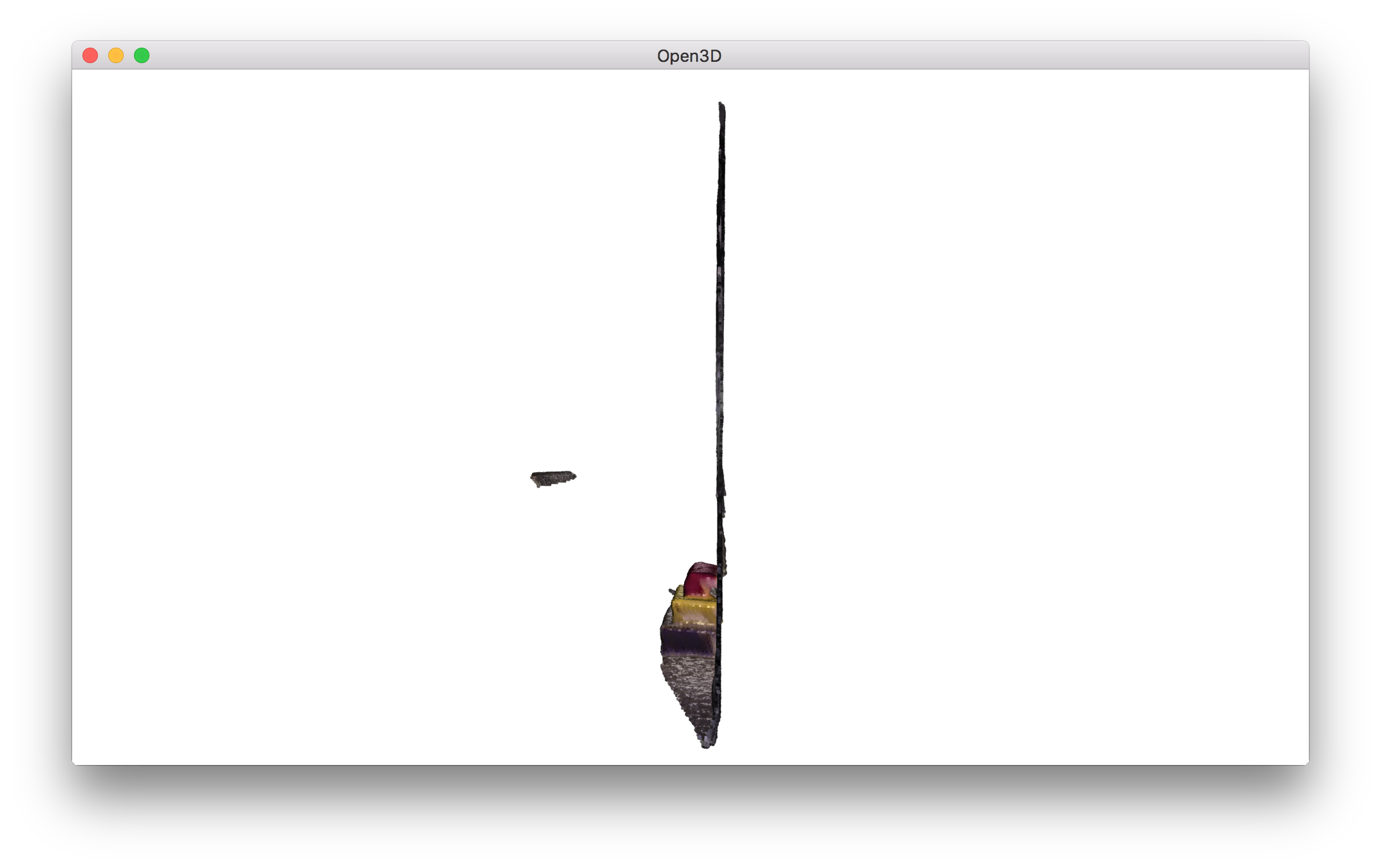# Colored point cloud registration¶

This tutorial demonstrates an ICP variant that uses both geometry and color for registration. It implements the algorithm of [Park2017]. The color information locks the alignment along the tangent plane. Thus this algorithm is more accurate and more robust than prior point cloud registration algorithms, while the running speed is comparable to that of ICP registration. This tutorial uses notations from ICP registration.

  5 6 7 8 9 10 11 12 13 14 15 16 17 18 19 20 21 22 23 24 25 26 27 28 29 30 31 32 33 34 35 36 37 38 39 40 41 42 43 44 45 46 47 48 49 50 51 52 53 54 55 56 57 58 59 60 61 62 63 64 65 66 67 68 69 70 71 72 # examples/Python/Advanced/colored_pointcloud_registration.py import numpy as np import copy import open3d as o3d def draw_registration_result_original_color(source, target, transformation): source_temp = copy.deepcopy(source) source_temp.transform(transformation) o3d.visualization.draw_geometries([source_temp, target]) if __name__ == "__main__": print("1. Load two point clouds and show initial pose") source = o3d.io.read_point_cloud("../../TestData/ColoredICP/frag_115.ply") target = o3d.io.read_point_cloud("../../TestData/ColoredICP/frag_116.ply") # draw initial alignment current_transformation = np.identity(4) draw_registration_result_original_color(source, target, current_transformation) # point to plane ICP current_transformation = np.identity(4) print("2. Point-to-plane ICP registration is applied on original point") print(" clouds to refine the alignment. Distance threshold 0.02.") result_icp = o3d.registration.registration_icp( source, target, 0.02, current_transformation, o3d.registration.TransformationEstimationPointToPlane()) print(result_icp) draw_registration_result_original_color(source, target, result_icp.transformation) # colored pointcloud registration # This is implementation of following paper # J. Park, Q.-Y. Zhou, V. Koltun, # Colored Point Cloud Registration Revisited, ICCV 2017 voxel_radius = [0.04, 0.02, 0.01] max_iter = [50, 30, 14] current_transformation = np.identity(4) print("3. Colored point cloud registration") for scale in range(3): iter = max_iter[scale] radius = voxel_radius[scale] print([iter, radius, scale]) print("3-1. Downsample with a voxel size %.2f" % radius) source_down = source.voxel_down_sample(radius) target_down = target.voxel_down_sample(radius) print("3-2. Estimate normal.") source_down.estimate_normals( o3d.geometry.KDTreeSearchParamHybrid(radius=radius * 2, max_nn=30)) target_down.estimate_normals( o3d.geometry.KDTreeSearchParamHybrid(radius=radius * 2, max_nn=30)) print("3-3. Applying colored point cloud registration") result_icp = o3d.registration.registration_colored_icp( source_down, target_down, radius, current_transformation, o3d.registration.ICPConvergenceCriteria(relative_fitness=1e-6, relative_rmse=1e-6, max_iteration=iter)) current_transformation = result_icp.transformation print(result_icp) draw_registration_result_original_color(source, target, result_icp.transformation) 

## Helper visualization function¶

 12 13 14 15 def draw_registration_result_original_color(source, target, transformation): source_temp = copy.deepcopy(source) source_temp.transform(transformation) o3d.visualization.draw_geometries([source_temp, target]) 

In order to demonstrate the alignment between colored point clouds, draw_registration_result_original_color renders point clouds with their original color.

## Input¶

 20 21 22 23 24 25 26 27  print("1. Load two point clouds and show initial pose") source = o3d.io.read_point_cloud("../../TestData/ColoredICP/frag_115.ply") target = o3d.io.read_point_cloud("../../TestData/ColoredICP/frag_116.ply") # draw initial alignment current_transformation = np.identity(4) draw_registration_result_original_color(source, target, current_transformation) 

This script reads a source point cloud and a target point cloud from two files. An identity matrix is used as initialization.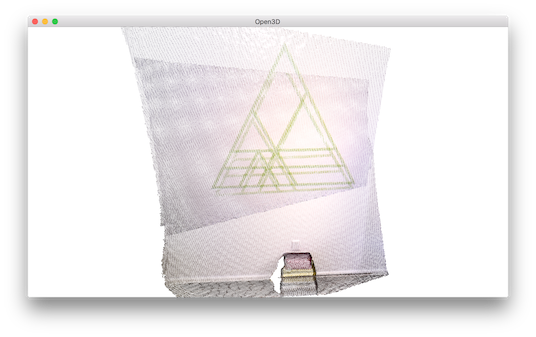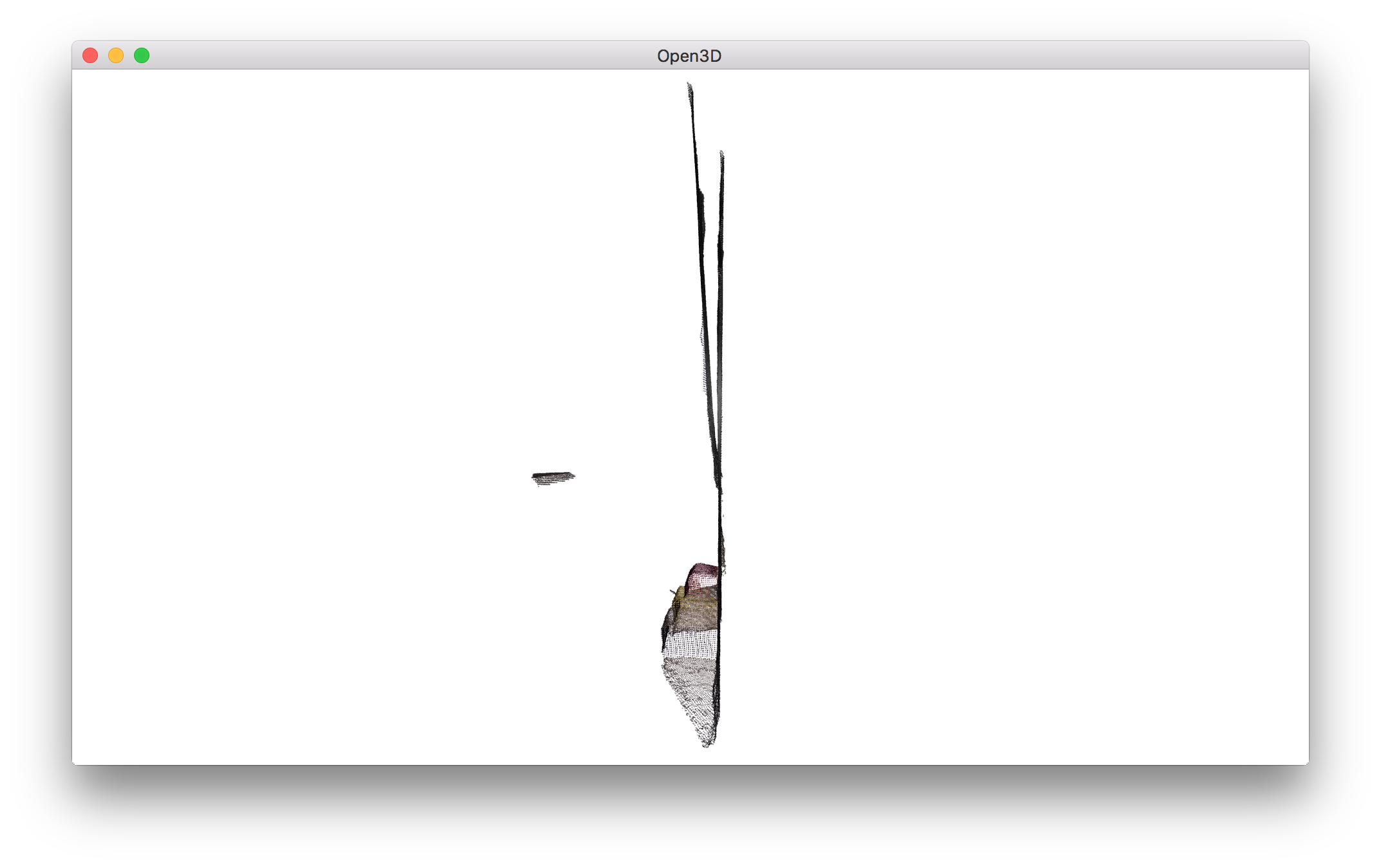## Point-to-plane ICP¶

 29 30 31 32 33 34 35 36 37 38  # point to plane ICP current_transformation = np.identity(4) print("2. Point-to-plane ICP registration is applied on original point") print(" clouds to refine the alignment. Distance threshold 0.02.") result_icp = o3d.registration.registration_icp( source, target, 0.02, current_transformation, o3d.registration.TransformationEstimationPointToPlane()) print(result_icp) draw_registration_result_original_color(source, target, result_icp.transformation) 

We first run Point-to-plane ICP as a baseline approach. The visualization below shows misaligned green triangle textures. This is because geometric constraint does not prevent two planar surfaces from slipping.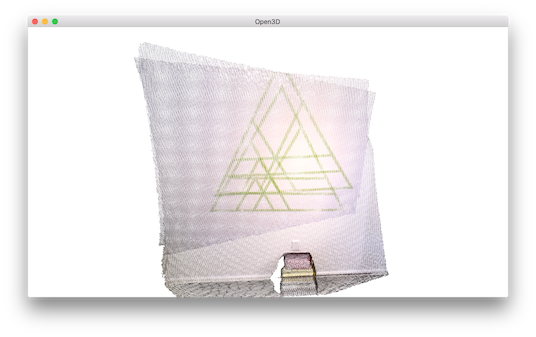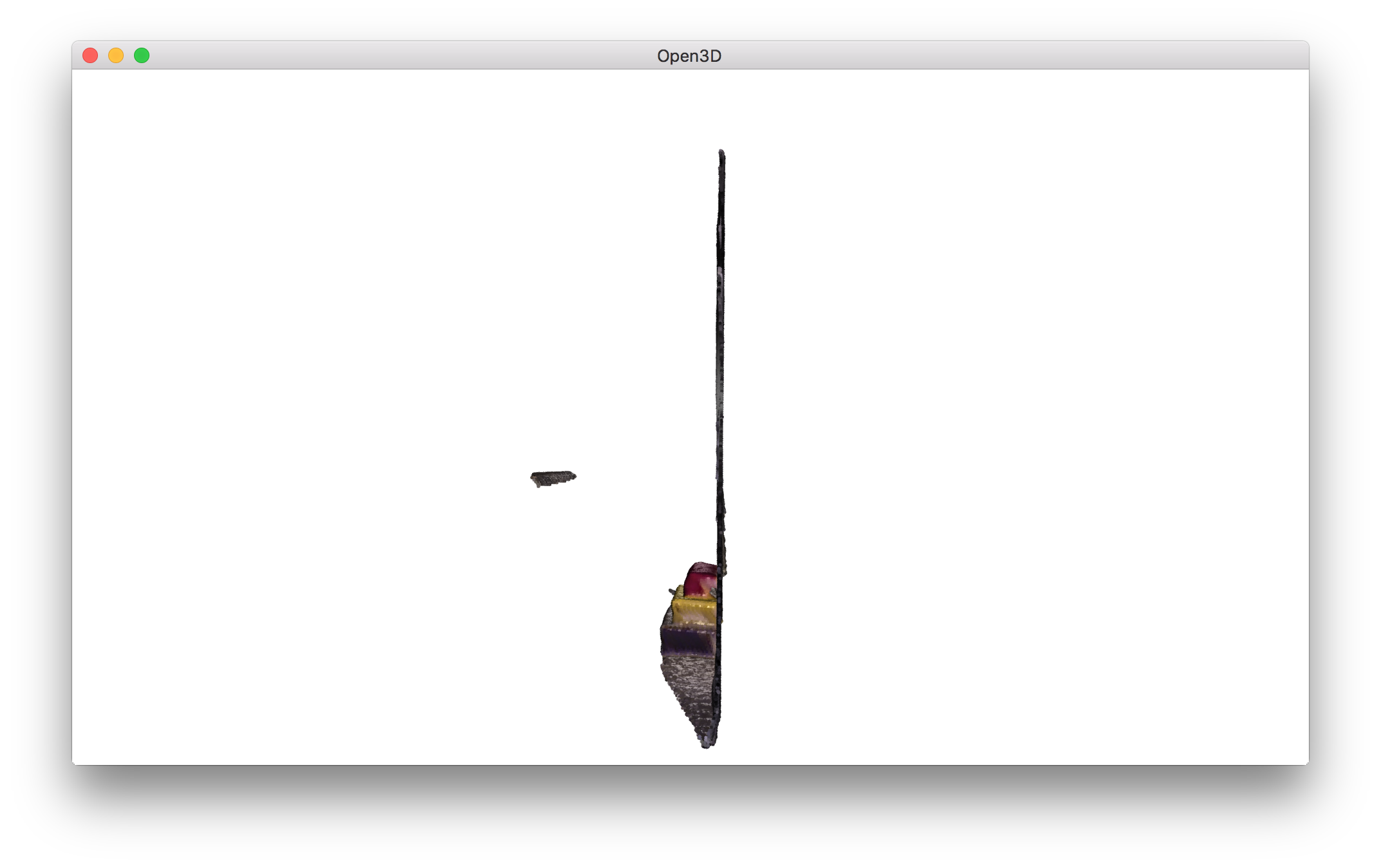## Colored point cloud registration¶

The core function for colored point cloud registration is registration_colored_icp. Following [Park2017], it runs ICP iterations (see Point-to-point ICP for details) with a joint optimization objective

$E(\mathbf{T}) = (1-\delta)E_{C}(\mathbf{T}) + \delta E_{G}(\mathbf{T}),$

where $$\mathbf{T}$$ is the transformation matrix to be estimated. $$E_{C}$$ and $$E_{G}$$ are the photometric and geometric terms, respectively. $$\delta\in[0,1]$$ is a weight parameter that has been determined empirically.

The geometric term $$E_{G}$$ is the same as the Point-to-plane ICP objective

$E_{G}(\mathbf{T}) = \sum_{(\mathbf{p},\mathbf{q})\in\mathcal{K}}\big((\mathbf{p} - \mathbf{T}\mathbf{q})\cdot\mathbf{n}_{\mathbf{p}}\big)^{2},$

where $$\mathcal{K}$$ is the correspondence set in the current iteration. $$\mathbf{n}_{\mathbf{p}}$$ is the normal of point $$\mathbf{p}$$.

The color term $$E_{C}$$ measures the difference between the color of point $$\mathbf{q}$$ (denoted as $$C(\mathbf{q})$$) and the color of its projection on the tangent plane of $$\mathbf{p}$$.

$E_{C}(\mathbf{T}) = \sum_{(\mathbf{p},\mathbf{q})\in\mathcal{K}}\big(C_{\mathbf{p}}(\mathbf{f}(\mathbf{T}\mathbf{q})) - C(\mathbf{q})\big)^{2},$

where $$C_{\mathbf{p}}(\cdot)$$ is a precomputed function continuously defined on the tangent plane of $$\mathbf{p}$$. Function $$\mathbf{f}(\cdot)$$ projects a 3D point to the tangent plane. More details refer to [Park2017].

To further improve efficiency, [Park2017] proposes a multi-scale registration scheme. This has been implemented in the following script.

 40 41 42 43 44 45 46 47 48 49 50 51 52 53 54 55 56 57 58 59 60 61 62 63 64 65 66 67 68 69 70 71 72  # colored pointcloud registration # This is implementation of following paper # J. Park, Q.-Y. Zhou, V. Koltun, # Colored Point Cloud Registration Revisited, ICCV 2017 voxel_radius = [0.04, 0.02, 0.01] max_iter = [50, 30, 14] current_transformation = np.identity(4) print("3. Colored point cloud registration") for scale in range(3): iter = max_iter[scale] radius = voxel_radius[scale] print([iter, radius, scale]) print("3-1. Downsample with a voxel size %.2f" % radius) source_down = source.voxel_down_sample(radius) target_down = target.voxel_down_sample(radius) print("3-2. Estimate normal.") source_down.estimate_normals( o3d.geometry.KDTreeSearchParamHybrid(radius=radius * 2, max_nn=30)) target_down.estimate_normals( o3d.geometry.KDTreeSearchParamHybrid(radius=radius * 2, max_nn=30)) print("3-3. Applying colored point cloud registration") result_icp = o3d.registration.registration_colored_icp( source_down, target_down, radius, current_transformation, o3d.registration.ICPConvergenceCriteria(relative_fitness=1e-6, relative_rmse=1e-6, max_iteration=iter)) current_transformation = result_icp.transformation print(result_icp) draw_registration_result_original_color(source, target, result_icp.transformation) 

In total, 3 layers of multi-resolution point clouds are created with Voxel downsampling. Normals are computed with Vertex normal estimation. The core registration function registration_colored_icp is called for each layer, from coarse to fine. lambda_geometric is an optional argument for registration_colored_icp that determines $$\lambda \in [0,1]$$ in the overall energy $$\lambda E_{G} + (1-\lambda) E_{C}$$.

The output is a tight alignment of the two point clouds. Notice the green triangles on the wall.